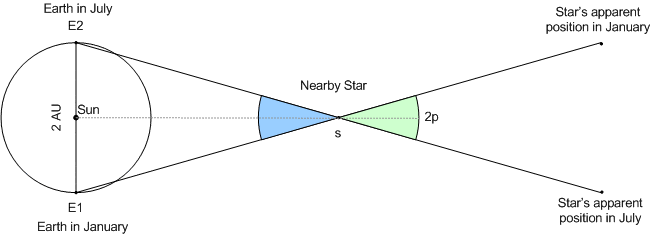# Parallax method

• B
iam in class 11th iam suffering from a great difficulty to understand parallex method , inspite of book's example

phinds
Gold Member
2021 Award
iam in class 11th iam suffering from a great difficulty to understand parallex method , inspite of book's example
Explain what you think the parallax (not "parallex") method is and what it does. That is, tell us how you see it and be more explicit about what you aren't following in the explanation in your book.

In the parallax method you look at something(prabably a star) from different positions.
The trick is that an approximately infinitely far away object does not seem to move anywhere.
Even if you change your position.
For example you don't see the sun move in response to your movement.
It stays where it is in the sky, no matter where you are or how you move.
(assuming you don't move somewhere entirely different on the planet)
The thing you are looking at however does move if you change your position.
You will see that the finger seems to change its position relative to the background.
By examining how much its position changes you can determine the distance you are away from it.
http://lcogt.net/files/styles/fourcol-image/public/spacebook/Parallax%20schematic.png [Broken]
In astronomy the "moving your head" is done by looking at the object from two opposite points on the earths orbit.

You might be able to see that the parallax angle does not only appear where it is drawn in but it is also half the difference in apparent position in the object.
So by measuring the angle and because we know the diameter of the earths orbit we can determine the distance from the star.

Last edited by a moderator:
•rtd
will you please tell me a solved example ?

Well i have never solved one of these ...
But i am gonna give it a try.
## tan(\Phi)=\frac {r}{d} ##
would make sense to me
(it might be wrong)

Phi is the parallax angle drawn in in the picture
r is the radius of the earths orbit
d is the distance to the star

solve it and you're done

thank u so much

Remember it might be wrong ....
Especially since the earths orbit is elliptical.(that makes the "radius" thing an approximation )
And you'll have to be careful to check whether your parallax angle is the one drawn in or the difference in position of the star.(that is just twice the angle that has been drawn in) Then you use twice the radius or just the diameter of the earths orbit

okkk

ok
The equation seems to be right...You see the parallax angle is always half the angle between the position of the stars.(that would be the angles drawn in in this one)
so dont worry about that thing with the angle.